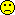## Who can help? [🇷 for BE/BA]

Important update, I forgot vBR on the diagonal. Now the singuality is less of a heacahe:

 rm(list=ls(all=TRUE)) ###### par vector is c(varT, varBR, varWR) ###### ######   Section 1: Household things ###### CreateX=function(D) {  ##first the two treatments  TrtT=as.numeric(as.character(D$Trt)=="T") TrtR=as.numeric(as.character(D$Trt)=="R")  X=cbind(TrtT, TrtR)  R= qr(X)$rank ## subjects: in a mixed model with subj as random ## we do not do subjects also as fixed, therefore they are #'ed away here ## for (s in unique(D$Subj))  ## {  ## v=as.numeric(D$Subj==s) ## #print(v) ## XX=data.frame(X, v) ## names(XX)[ncol(XX)] = paste("S", s, sep="") ## rnk=qr(XX)$rank  ## if (rnk>R)   ##  {   ##     X=XX   ##     R=rnk   ##  }   ## }  ##now the Pers  for (p in unique(D$Per)) { v=as.numeric(D$Per==p)   #print(v)   XX=data.frame(X, v)   names(XX)[ncol(XX)] = paste("P", p, sep="")   rnk=qr(XX)$rank if (rnk>R) { X=XX R=rnk } } for (q in unique(D$Seq))  {   v=as.numeric(D$Seq==q) #print(v) XX=data.frame(X, v) names(XX)[ncol(XX)] = paste("Q", q, sep="") rnk=qr(XX)$rank   if (rnk>R)     {        X=XX        R=rnk     }  }  return(as.matrix(X)) } Create.CovM=function(Params) ##block diagonal covariance matrix {   varT=Params   varBR=Params   varWR=Params   #varRT=Params   #cat("Vars:", varT, varBR, varWR,"\n")   Nobs=length(D$Y) V=matrix(0,ncol=Nobs, nrow=Nobs) for (iRow in 1:Nobs) for (iCol in 1:Nobs) { ## the diagonal if (iCol==iRow) { if (D$Trt[iRow]=="T") V[iRow,iCol]=V[iRow,iCol]+varT       if (D$Trt[iRow]=="R") V[iRow,iCol]=V[iRow,iCol]+varWR +varBR } ## off diagonal if (iCol!=iRow) if (D$Subj[iRow]==D$Subj[iCol]) { if (D$Trt[iCol]==D$Trt[iRow]) V[iRow,iCol]= V[iRow,iCol]+varBR #if (D$Trt[iCol]!=D$Trt[iRow]) V[iRow,iCol]= V[iRow,iCol]+varRT } } return(as.matrix(V)) } ###### ###### Section 2: Matrix things ###### Obj.F12=function(Pars) ##this is line 3 of page 10 of: ##http://people.csail.mit.edu/xiuming/docs/tutorials/reml.pdf { CovM=Create.CovM(Pars) A= -0.5*log(det(CovM)) B= -0.5*log(det(t(X) %*% solve(CovM) %*% X)) est.b = solve(t(X) %*% solve(CovM) %*% X) %*% t(X) %*% solve(CovM) %*% y tmp= y - X %*% est.b C=-0.5 *(t(tmp) %*% solve(CovM) %*% tmp) return(A+B+C) } Some.Initial.Guesses=function(foo) { #guess variance within R D1=subset(D, D$Trt=="R")   m=lm(log(Y)~factor(Subj)+factor(Seq)+factor(Per) , data=D1)   #print(anova(m))   varWR= anova(m)["Residuals", "Mean Sq"]   m=lm(log(Y)~factor(Subj)+factor(Seq)+factor(Per)+factor(Trt) , data=D)   varBR= anova(m)["factor(Subj)", "Mean Sq"]   ##total var T, cheaply   ##actually, this may be much better: varT=varBR+varWR   D2=subset(D, D$Trt=="T") varT=var(log(D2$Y))   varT=varBR+varWR   L=list(varT=varT, varBR=varBR, varWR=varWR)   return(L) } #### #### section 3: execution #### D=read.csv("EMAII.a.csv" , header=T, sep="\t") ##public = easier X=CreateX(D) ##public = easier y=log(D$Y) ##public = easier Check.Surf=function(i, x1,x2, N) ## Checks the log likelihood surface of the i'th variance component ## (given the other variance components) for the interval var = x1 to x2 ## with a granularity of N points. { L=Some.Initial.Guesses(0) parIni=c(L$varT, L$varBR, L$varWR )   x=x1;   vX=NULL   vY=NULL   dx=(x2-x1)/N   for (j in 1:N)   {     vX=c(vX, x)     parIni[i]=x     vY=c(vY, Obj.F12(parIni))     x=x+dx   }   plot(vX, vY) } MyREML=function(foo.bar) ## ideally this would work, but it doesn't right now## also, I have a feeling the surface may be quite rugged, ## so I may switch to a homecooked optimiser ## once I have ironed out the singularity issue, see below. {   L=Some.Initial.Guesses(0)   print(L)   parIni=c(L$varT, L$varBR, L\$varWR)   F=optim(par=parIni, fn=Obj.F12, #method="L-BFGS-B",lower=c(0,0,0),       control=list(reltol=1e-10, trace=T))   print(F) } # if ti worked it'd be called through  MyREML(0) # however: Check.Surf(3, 0.01, 0.2, 50) ##checks the surface in one dimension # # # 

Pass or fail!
ElMaestroIng. Helmut Schütz